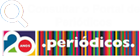Navegar por:

## Correspondence between the one-loop three-point vertex and the Y and Delta electric resistor networks

Autor(es): dc.creatorSuzuki, Alfredo Takashi-
Data de aceite: dc.date.accessioned2021-03-10T21:21:24Z-
Data de disponibilização: dc.date.available2021-03-10T21:21:24Z-
Data de envio: dc.date.issued2014-12-03-
Data de envio: dc.date.issued2014-12-03-
Data de envio: dc.date.issued2014-02-01-
Fonte completa do material: dc.identifierhttp://dx.doi.org/10.1139/cjp-2012-0403-
Fonte completa do material: dc.identifierhttp://hdl.handle.net/11449/113050-
Fonte: dc.identifier.urihttp://educapes.capes.gov.br/handle/11449/113050-
Descrição: dc.descriptionDifferent mathematical methods have been applied to obtain the analytic result for the massless triangle Feynman diagram yielding a sum of four linearly independent (LI) hypergeometric functions of two variables F-4. This result is not physically acceptable when it is embedded in higher loops, because all four hypergeometric functions in the triangle result have the same region of convergence and further integration means going outside those regions of convergence. We could go outside those regions by using the well-known analytic continuation formulas obeyed by the F-4, but there are at least two ways we can do this. Which is the correct one? Whichever continuation one uses, it reduces a number of F-4 from four to three. This reduction in the number of hypergeometric functions can be understood by taking into account the fundamental physical constraint imposed by the conservation of momenta flowing along the three legs of the diagram. With this, the number of overall LI functions that enter the most general solution must reduce accordingly. It remains to determine which set of three LI solutions needs to be taken. To determine the exact structure and content of the analytic solution for the three-point function that can be embedded in higher loops, we use the analogy that exists between Feynman diagrams and electric circuit networks, in which the electric current flowing in the network plays the role of the momentum flowing in the lines of a Feynman diagram. This analogy is employed to define exactly which three out of the four hypergeometric functions are relevant to the analytic solution for the Feynman diagram. The analogy is built based on the equivalence between electric resistance circuit networks of types Y and Delta in which flows a conserved current. The equivalence is established via the theorem of minimum energy dissipation within circuits having these structures.-
Formato: dc.format131-135-
Idioma: dc.languageen-